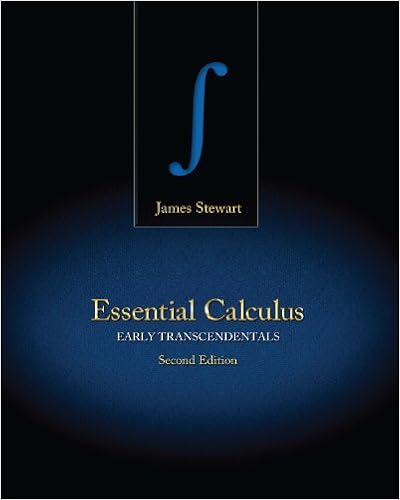# Rigid_Bodies_print - Chapter 6 Rigid Body Dynamics 6.1...

• 56

This preview shows page 1 - 2 out of 56 pages.

##### We have textbook solutions for you!
The document you are viewing contains questions related to this textbook.The document you are viewing contains questions related to this textbook.
Chapter 10 / Exercise 18
Essential Calculus: Early Transcendentals
StewartExpert Verified
Chapter 6 Rigid Body Dynamics 6.1 Introduction In practice, it is often not possible to idealize a system as a particle. In this section, we construct a more sophisticated description of the world, in which objects rotate , in addition to translating. This general branch of physics is called ‘Rigid Body Dynamics.’ Rigid body dynamics has many applications. In vehicle dynamics, we are often more worried about controlling the orientation of our vehicle than its path – an aircraft must keep its shiny side up, and we don’t want a spacecraft tumbling uncontrollably. Rigid body mechanics is used extensively to design power generation and transmission systems, from jet engines, to the internal combustion engine, to gearboxes. A typical problem is to convert rotational motion to linear motion, and vice-versa. Rigid body motion is also of great interest to people who design prosthetic devices, implants, or coach athletes: here, the goal is to understand human motion, to protect athletes from injury or improve their performance, or to design devices that replicate the complicated motion of a human joint correctly. For example, Professor Crisco’s orthopaedics lab at Brown studies human motion and the forces they generate at human joints, to help understand how injuries occur and how they can be prevented. The motion of a rigid body is often very counter-intuitive. That’s why there are so many toys that exploit the properties of rigid bodies: the motion of a spinning top; a boomerang; the ‘rattleback’ and a Frisbee can all be explained using the equations derived in this section. Here is a quick outline of how we analyze motion of rigid bodies. 1. A rigid body is idealized as an infinite number of very small particles. 2. We already know the equations of motion for a system of particles (Section 4 of the notes): The force-momentum equation 1 N ext i i i i i d d m dt dt = = = p F v The moment – angular momentum equation 1 N ext i i i i i i i d d m dt dt = × = = × h r F r v The work-kinetic energy equation 1 1 2 N ext i i i i i i i dT d m dt dt = = = F v v v 3. These equations tell us how a rigid body moves. But to use them, we would need to keep track track of an infinite number of particles! To simplify the problem, we set up some mathematical methods that allow us to express the position and velocity of every point in a rigid body in terms of the position G r , velocity G v and acceleration G a of its center of mass, and its rotation tensor R (quantifying its orientation) and its angular velocity ω , and angular acceleration α . This allows us to write the linear momentum, angular momentum, and kinetic energy of a rigid body in the form G M = p v G G G M = × + h r v I ω 1 1 2 2 G G G T M = + v v ω I ω where M is the total mass of the body and G I is its mass moment of inertia.
##### We have textbook solutions for you!
The document you are viewing contains questions related to this textbook.The document you are viewing contains questions related to this textbook.
Chapter 10 / Exercise 18
Essential Calculus: Early Transcendentals
StewartExpert Verified
•••